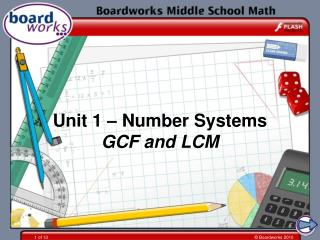# Unit 1 – Number Systems GCF and LCM - PowerPoint PPT PresentationDownload PresentationUnit 1 – Number Systems GCF and LCM

Unit 1 – Number Systems GCF and LCMDownload Presentation## Unit 1 – Number Systems GCF and LCM

- - - - - - - - - - - - - - - - - - - - - - - - - - - E N D - - - - - - - - - - - - - - - - - - - - - - - - - - -
##### Presentation Transcript

1. Unit 1 – Number SystemsGCF and LCM

2. Common multiples Multiples of 6 Multiples of 8 12 60 6 18 54 66 102… 24 24 48 48 96 96 72 8 16 32 40 56 64 72 80 88 104 … 30 42 78 90 84 36

3. Multiples on a hundred square

4. The least common multiple The least common multiple (or LCM) of two numbers is the smallest number that is a multiple of both the numbers. We can find this by writing down the first few multiples for both numbers until we find a number that is in both lists. For example: 100, Multiples of 20 are : 20, 40, 60, 80, 120, . . . 25, 50, 75, 100, 125, . . . Multiples of 25 are : The LCM of 20 and 25 is 100.

5. The least common multiple What is the least common multiple (LCM) of 8 and 10? The first ten multiples of 8 are: 8, 16, 24, 32, 40, 48, 56, 64, 72, 80. The first ten multiples of 10 are: 10, 20, 30, 40, 50, 60, 70, 80, 90, 100. The least common multiple (LCM) of 8 and 10 is 40.

6. The least common multiple 5 5 and . Add together 4 4 12 12 9 9 × 4 × 3 31 + = + 36 36 36 × 4 × 3 We use the least common multiplewhen adding and subtracting fractions. The LCM of 9 and 12 is 36. 16 15 =

7. Common factor diagram

8. The greatest common factor The greatest common factor (or GCF) of two numbers is the largest number that is a factor of both numbers. We can find the greatest common factor of two numbers by writing down all their factors and finding the largest factor in both lists. For example: Factors of 36 are : 1, 2, 3, 4, 6, 9, 12, 18, 36. Factors of 45 are : 1, 3, 5, 9, 15, 45. The GCF of 36 and 45 is 9.

9. The greatest common factor What is the greatest common factor (GCF) of 24 and 30? The factors of 24 are: 1, 2, 3, 4, 6, 8, 12, 24. The factors of 30 are: 1, 2, 3, 5, 6, 10, 15, 30. The greatest common factor (GCF) of 24 and 30 is 6.

10. The greatest common factor 36 36 48 48 ÷12 ÷12 We use the greatest common factor when simplifying fractions. Simplify the fraction . The GCF of 36 and 48 is 12, so we need to divide the numerator and the denominator by 12. 3 = 4

11. Using prime factors to find the GCF and LCM 2 60 2 294 1 1 We can use the prime factorization to find the GCF and LCM of larger numbers. Find the GCF and the LCM of 60 and 294. 2 30 3 147 3 15 7 49 5 5 7 7 60 = 2 × 2 × 3 × 5 294 = 2 × 3 × 7 × 7

12. Using prime factors to find the GCF and LCM 60 = 2 × 2 × 3 × 5 294 = 2 × 3 × 7 × 7 60 294 2 7 2 3 5 7 GCF of 60 and 294 = 2 × 3 = 6 LCM of 60 and 294 = 2 × 5 × 2 × 3 × 7 × 7 = 2940

13. Using prime factors to find the GCF and LCM